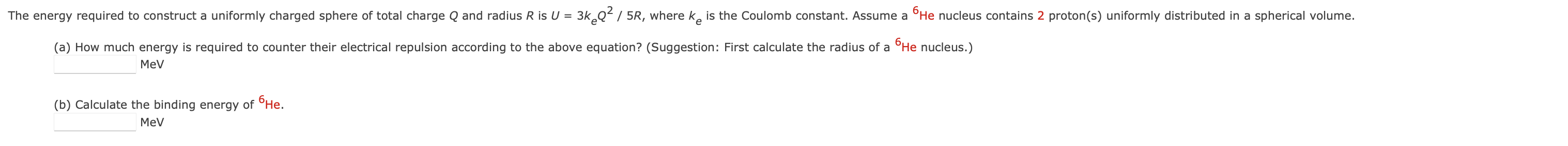Home / Expert Answers / Physics / a-how-much-energy-is-required-to-counter-their-electrical-repulsion-according-to-the-above-equat-pa445

# (Solved): (a) How much energy is required to counter their electrical repulsion according to the above equat ...(a) How much energy is required to counter their electrical repulsion according to the above equation? (Suggestion: First calculate the radius of a He nucleus.) (b) Calculate the binding energy of .

We have an Answer from Expert

Given,
Number of protons in   nucleus

a) To find the energy required to counter the electric repulsion.

Here,   - Coulomb's constant(  ,   - total charge and   - radius of the helium nucleus.

Here,    is calculated by,

Here,   - radius of a nucleon (  ) and    - atomic number
We have an Answer from Expert